Premium

# 3.MD.C.7 Worksheets, Workbooks, Lesson Plans, and Games

#### CCSS.maths.CONTENT.3.MD.C.7

:
"Relate area to the operations of multiplication and addition."

These worksheets can help students practise this Common Core State Standards skill.

## Worksheets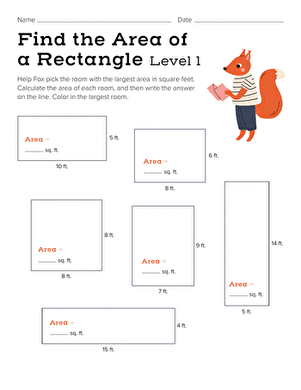Find the Area of a Rectangle: Easy
Worksheet
Find the Area of a Rectangle: Easy
Piggy needs to find a big room to house his teddy bear collection. Can your child help him figure out which room is the largest?
Year 5
Maths
Worksheet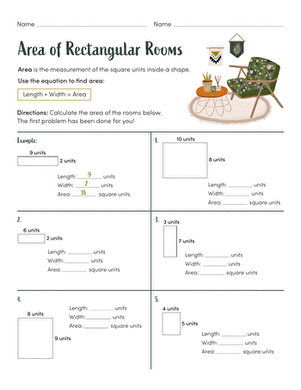Area of Rectangular Rooms
Worksheet
Area of Rectangular Rooms
In this exercise, students will practise finding the area of a rectangle.
Year 4
Maths
Worksheet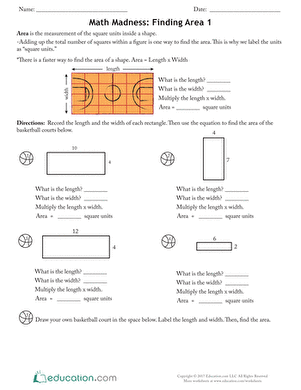Maths Madness: Finding Area 1
Worksheet
Maths Madness: Finding Area 1
With this maths Madness exercise, they will find the area of different basketball courts.
Year 4
Maths
Worksheet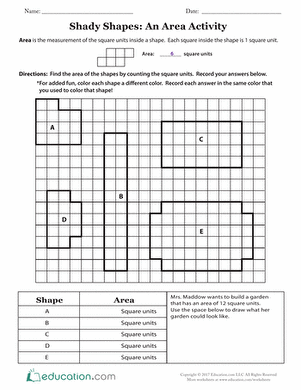Shady Shapes: An Area Activity
Worksheet
Shady Shapes: An Area Activity
Use this fun exercise with your students to practise finding the area of shapes by counting unit squares.
Year 4
Maths
Worksheet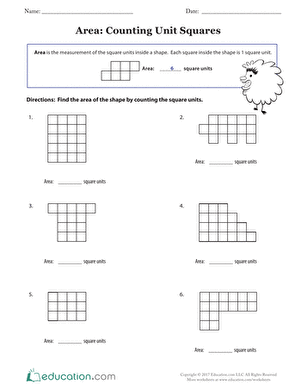Area: Counting Unit Squares
Worksheet
Area: Counting Unit Squares
Use this exercise with your students to build a foundational understanding of geometry. They will determine the area of shapes by counting the unit squares.
Year 4
Maths
Worksheet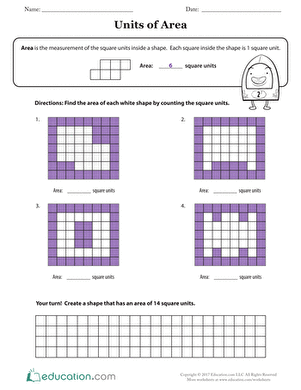Units of Area
Worksheet
Units of Area
Build your students’ foundational understanding of geometry as they determine the area of shapes by counting the unit squares.
Year 4
Maths
Worksheet

## Lesson Plans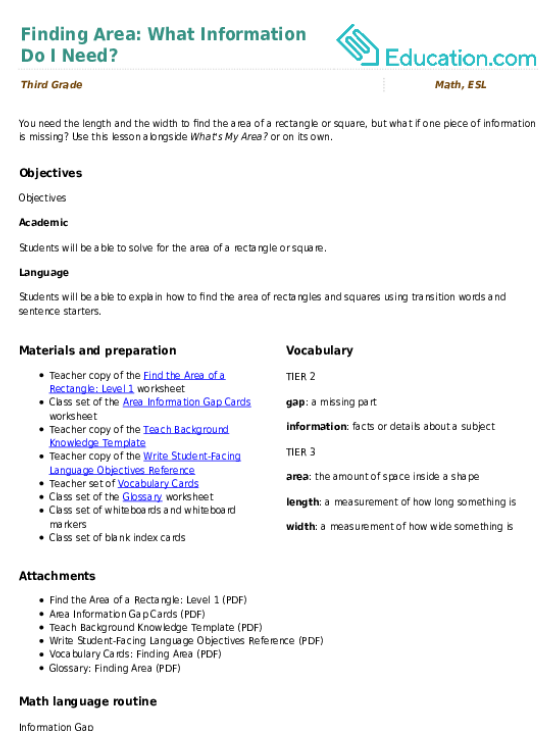Finding Area: What Information Do I Need?
Lesson plan
Finding Area: What Information Do I Need?
You need the length and the width to find the area of a rectangle or square, but what if one piece of information is missing? Use this lesson alongside *What's My Area?* or on its own.
Year 4
Maths
Lesson plan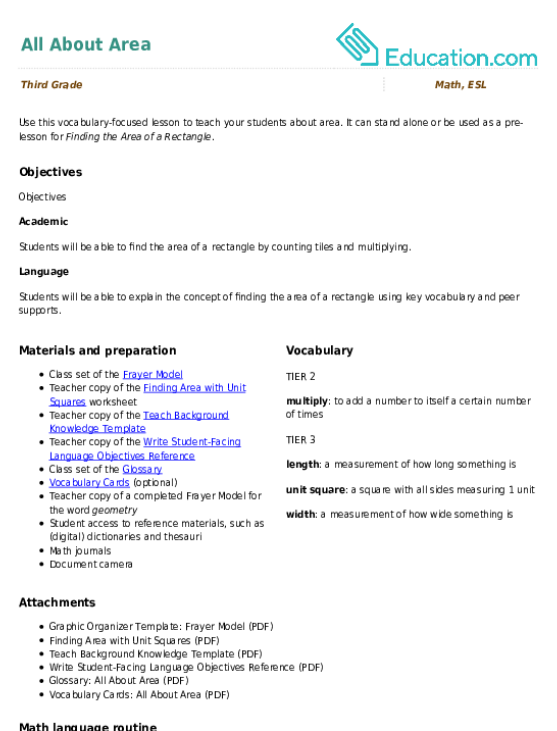All About Area
Lesson plan
All About Area
Use this vocabulary-focused lesson to teach your students about area. It can stand alone or be used as a pre-lesson for *Finding the Area of a Rectangle*.
Year 4
Maths
Lesson plan

## Workbooks

No workbooks found for this common core node.

## Games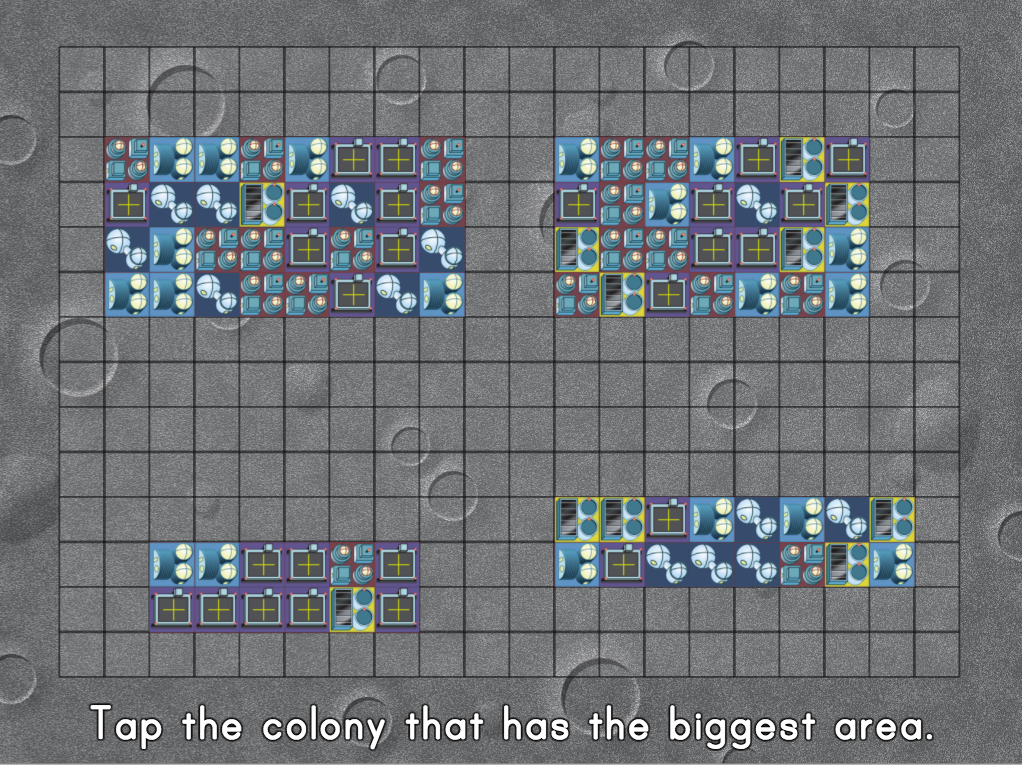Colony Area Measurement
Game
Colony Area Measurement
In this space-themed game, kids find the area of new colonies by using repeated addition and multiplication strategies.
Year 4
Maths
Game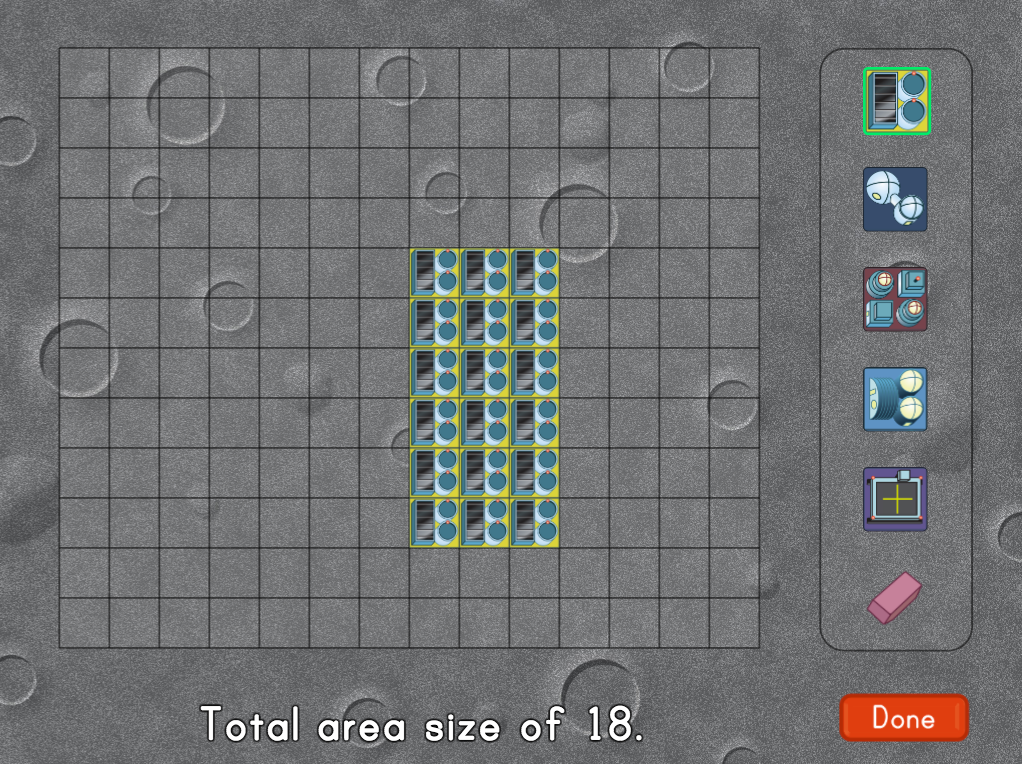City Building: Square Unit practise
Game
City Building: Square Unit practise
Kids learn that area is calculated in square units through this interactive space colony building game.
Year 4
Maths
Game

## Exercises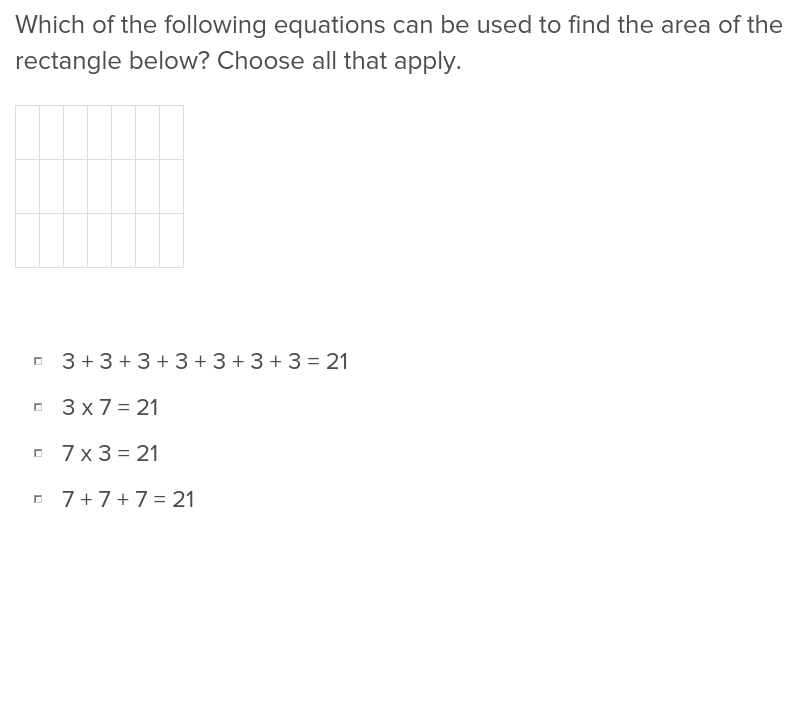Relating Area to Addition and Multiplication
Exercise
Relating Area to Addition and Multiplication
Help students understand the relation between addition and multiplication, and the area of objects, with this easy to navigate exercise.
Year 4
Maths
Exercise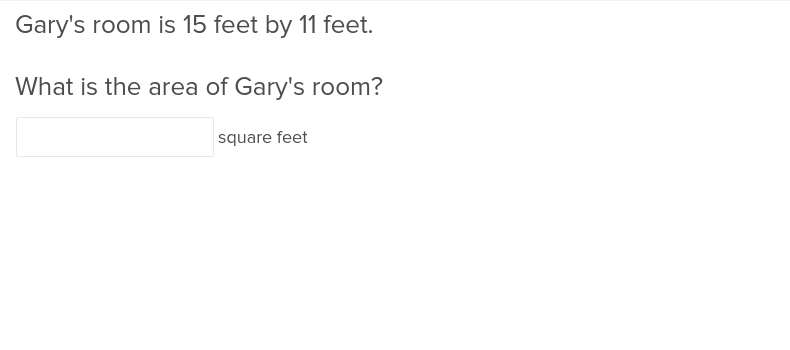Area of a Rectangle Using Square Units
Exercise
Area of a Rectangle Using Square Units
This exercise will students how to calculate the area of a rectangle with ease using square units.
Year 4
Maths
Exercise

### Add to collection

Create new collection

0

### New Collection>

0Items

What could we do to improve Education.com?

Please note: Use the Contact Us link at the bottom of our website for account-specific questions or issues.

What would make you love Education.com?